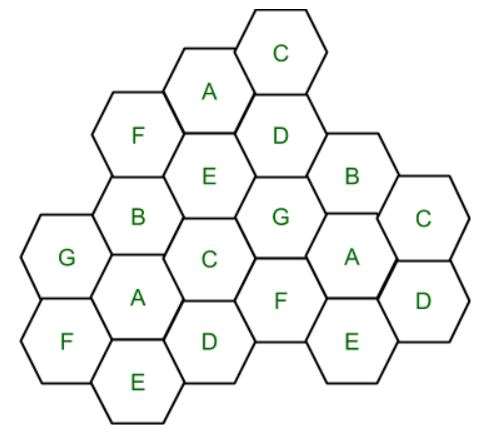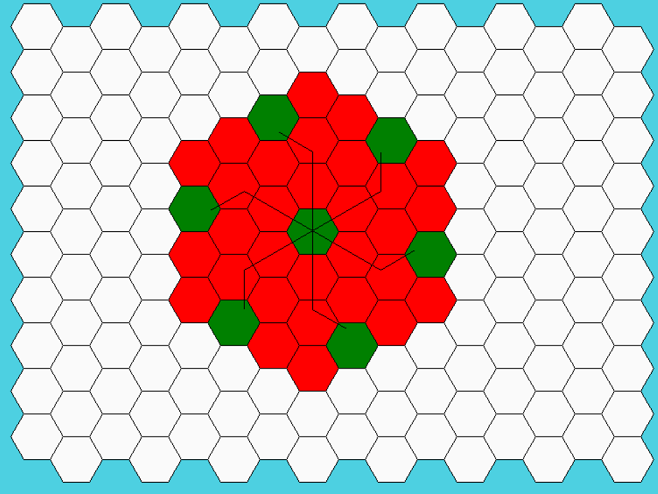# Frequency Reuse

• Difficulty Level : Medium
• Last Updated : 16 May, 2020

Frequency Reuse is the scheme in which allocation and reuse of channels throughout a coverage region is done. Each cellular base station is allocated a group of radio channels or Frequency sub-bands to be used within a small geographic area known as a cell. The shape of the cell is Hexagonal. The process of selecting and allocating the frequency sub-bands for all of the cellular base station within a system is called Frequency reuse or Frequency Planning.

Silent Features of using Frequency Reuse:

• Frequency reuse improve the spectral efficiency and signal Quality (QoS).
• Frequency reuse classical scheme proposed for GSM systems offers a protection against interference.
• The number of times a frequency can be reused is depend on the tolerance capacity of the radio channel from the nearby transmitter that is using the same frequencies.
• In Frequency Reuse scheme, total bandwidth is divided into different sub-bands that are used by cells.
• Frequency reuse scheme allow WiMax system operators to reuse the same frequencies at different cell sites.Cell with the same letter uses the same set of channels group or frequencies sub-band.

To find the total number of channel allocated to a cell:

S = Total number of duplex channels available to use
k = Channels allocated to each cell (k<S)
N = Total number of cells or Cluster Size

Then Total number of channels (S) will be,

```S = kN

Frequency Reuse Factor = 1/N```

In the above diagram cluster size is 7 (A,B,C,D,E,F,G) thus frequency reuse factor is 1/7.

N is the number of cells which collectively use the complete set of available frequencies is called a Cluster. The value of N is calculated by the following formula:

`N = I2 + I*J + J2 `

Where I,J = 0,1,2,3…
Hence, possible values of N are 1,3,4,7,9,12,13,16,19 and so on.

If a Cluster is replicated or repeated M times within the cellular system, then Capacity, C, will be,

`C = MkN = MS`

In Frequency reuse there are several cells that use the same set of frequencies. These cells are called Co-Channel Cells. These Co-Channel cells results in interference. So to avoid the Interference cells that use the same set of channels or frequencies are separated from one another by a larger distance. The distance between any two Co-Channels can be calculated by the following formula:

` D = R * (3 * N)1/2`

Where,
R = Radius of a cell
N = Number of cells in a given cluster

Below is the Python code for visualizing the Frequency Reuse concept

 `#!/usr/bin/python`` ` `from` `math ``import` `*`` ` `# import everything from Tkinter module``from` `tkinter ``import` `*`` ` `# Base class for Hexagon shape``class` `Hexagon(``object``):``    ``def` `__init__(``self``, parent, x, y, length, color, tags):``        ``self``.parent ``=` `parent``        ``self``.x ``=` `x``        ``self``.y ``=` `y``        ``self``.length ``=` `length``        ``self``.color ``=` `color``        ``self``.size ``=` `None``        ``self``.tags ``=` `tags``        ``self``.draw_hex()`` ` `    ``# draw one hexagon``    ``def` `draw_hex(``self``):``        ``start_x ``=` `self``.x``        ``start_y ``=` `self``.y``        ``angle ``=` `60``        ``coords ``=` `[]``        ``for` `i ``in` `range``(``6``):``            ``end_x ``=` `start_x ``+` `self``.length ``*` `cos(radians(angle ``*` `i))``            ``end_y ``=` `start_y ``+` `self``.length ``*` `sin(radians(angle ``*` `i))``            ``coords.append([start_x, start_y])``            ``start_x ``=` `end_x``            ``start_y ``=` `end_y``        ``self``.parent.create_polygon(coords[``0``][``0``],``                                ``coords[``0``][``1``],``                                ``coords[``1``][``0``],``                                ``coords[``1``][``1``],``                                ``coords[``2``][``0``],``                                ``coords[``2``][``1``],``                                ``coords[``3``][``0``],``                                ``coords[``3``][``1``],``                                ``coords[``4``][``0``],``                                ``coords[``4``][``1``],``                                ``coords[``5``][``0``],``                                ``coords[``5``][``1``],``                                ``fill``=``self``.color,``                                ``outline``=``"black"``,``                                ``tags``=``self``.tags)`` ` `# class holds frequency reuse logic and related methods``class` `FrequencyReuse(Tk):``    ``CANVAS_WIDTH ``=` `800``    ``CANVAS_HEIGHT ``=` `650``    ``TOP_LEFT ``=` `(``20``, ``20``)``    ``BOTTOM_LEFT ``=` `(``790``, ``560``)``    ``TOP_RIGHT ``=` `(``780``, ``20``)``    ``BOTTOM_RIGHT ``=` `(``780``, ``560``)`` ` `    ``def` `__init__(``self``, cluster_size, columns``=``16``, rows``=``10``, edge_len``=``30``):``        ``Tk.__init__(``self``)``        ``self``.textbox ``=` `None``        ``self``.curr_angle ``=` `330``        ``self``.first_click ``=` `True``        ``self``.reset ``=` `False``        ``self``.edge_len ``=` `edge_len``        ``self``.cluster_size ``=` `cluster_size``        ``self``.reuse_list ``=` `[]``        ``self``.all_selected ``=` `False``        ``self``.curr_count ``=` `0``        ``self``.hexagons ``=` `[]``        ``self``.co_cell_endp ``=` `[]``        ``self``.reuse_xy ``=` `[]``        ``self``.canvas ``=` `Canvas(``self``,``                            ``width``=``self``.CANVAS_WIDTH,``                            ``height``=``self``.CANVAS_HEIGHT,``                            ``bg``=``"#4dd0e1"``)``        ``self``.canvas.bind(``""``, ``self``.call_back)``        ``self``.canvas.focus_set()``        ``self``.canvas.bind(``''``, ``self``.resets)``        ``self``.canvas.pack()``        ``self``.title(``"Frequency reuse and co-channel selection"``)``        ``self``.create_grid(``16``, ``10``)``        ``self``.create_textbox()``        ``self``.cluster_reuse_calc()`` ` `    ``# show lines joining all co-channel cells``    ``def` `show_lines(``self``):``        ``# center(x,y) of first hexagon``        ``approx_center ``=` `self``.co_cell_endp[``0``]``        ``self``.line_ids ``=` `[]``        ``for` `k ``in` `range``(``1``, ``len``(``self``.co_cell_endp)):`` ` `            ``end_xx ``=` `(``self``.co_cell_endp[k])[``0``]``            ``end_yy ``=` `(``self``.co_cell_endp[k])[``1``]`` ` `            ``# move i^th steps``            ``l_id ``=` `self``.canvas.create_line(approx_center[``0``], approx_center[``1``],``                                        ``end_xx, end_yy)``            ``if` `j ``=``=` `0``:``                ``self``.line_ids.append(l_id)``                ``dist ``=` `0``            ``elif` `i >``=` `j ``and` `j !``=` `0``:``                ``self``.line_ids.append(l_id)``                ``dist ``=` `j``                ``# rotate counter-clockwise and move j^th step``                ``l_id ``=` `self``.canvas.create_line(``                    ``end_xx, end_yy, end_xx ``+` `self``.center_dist ``*` `dist ``*``                    ``cos(radians(``self``.curr_angle ``-` `60``)),``                    ``end_yy ``+` `self``.center_dist ``*` `dist ``*``                    ``sin(radians(``self``.curr_angle ``-` `60``)))``                ``self``.line_ids.append(l_id)``            ``self``.curr_angle ``-``=` `60`` ` `    ``def` `create_textbox(``self``):``        ``txt ``=` `Text(``self``.canvas,``                ``width``=``80``,``                ``height``=``1``,``                ``font``=``(``"Helvatica"``, ``12``),``                ``padx``=``10``,``                ``pady``=``10``)``        ``txt.tag_configure(``"center"``, justify``=``"center"``)``        ``txt.insert(``"1.0"``, ``"Select a Hexagon"``)``        ``txt.tag_add(``"center"``, ``"1.0"``, ``"end"``)``        ``self``.canvas.create_window((``0``, ``600``), anchor``=``'w'``, window``=``txt)``        ``txt.config(state``=``DISABLED)``        ``self``.textbox ``=` `txt`` ` `    ``def` `resets(``self``, event):``        ``if` `event.char ``=``=` `'R'``:``            ``self``.reset_grid()`` ` `    ``# clear hexagonal grid for new i/p``    ``def` `reset_grid(``self``, button_reset``=``False``):``        ``self``.first_click ``=` `True``        ``self``.curr_angle ``=` `330``        ``self``.curr_count ``=` `0``        ``self``.co_cell_endp ``=` `[]``        ``self``.reuse_list ``=` `[]``        ``for` `i ``in` `self``.hexagons:``            ``self``.canvas.itemconfigure(i.tags, fill``=``i.color)`` ` `        ``try``:``            ``self``.line_ids``        ``except` `AttributeError:``            ``pass``        ``else``:``            ``for` `i ``in` `self``.line_ids:``                ``self``.canvas.after(``0``, ``self``.canvas.delete, i)``            ``self``.line_ids ``=` `[]`` ` `        ``if` `button_reset:``            ``self``.write_text(``"Select a Hexagon"``)`` ` `    ``# create a grid of Hexagons``    ``def` `create_grid(``self``, cols, rows):``        ``size ``=` `self``.edge_len``        ``for` `c ``in` `range``(cols):``            ``if` `c ``%` `2` `=``=` `0``:``                ``offset ``=` `0``            ``else``:``                ``offset ``=` `size ``*` `sqrt(``3``) ``/` `2``            ``for` `r ``in` `range``(rows):``                ``x ``=` `c ``*` `(``self``.edge_len ``*` `1.5``) ``+` `50``                ``y ``=` `(r ``*` `(``self``.edge_len ``*` `sqrt(``3``))) ``+` `offset ``+` `15``                ``hx ``=` `Hexagon(``self``.canvas, x, y, ``self``.edge_len, ``"#fafafa"``,``                            ``"{},{}"``.``format``(r, c))``                ``self``.hexagons.append(hx)`` ` `    ``# calculate reuse distance, center distance and radius of the hexagon``    ``def` `cluster_reuse_calc(``self``):``        ``self``.hex_radius ``=` `sqrt(``3``) ``/` `2` `*` `self``.edge_len``        ``self``.center_dist ``=` `sqrt(``3``) ``*` `self``.hex_radius``        ``self``.reuse_dist ``=` `self``.hex_radius ``*` `sqrt(``3` `*` `self``.cluster_size)`` ` `    ``def` `write_text(``self``, text):``        ``self``.textbox.config(state``=``NORMAL)``        ``self``.textbox.delete(``'1.0'``, END)``        ``self``.textbox.insert(``'1.0'``, text, ``"center"``)``        ``self``.textbox.config(state``=``DISABLED)`` ` `    ``#check if the co-channels are within visible canvas``    ``def` `is_within_bound(``self``, coords):``        ``if` `self``.TOP_LEFT[``0``] < coords[``0``] < ``self``.BOTTOM_RIGHT[``0``] \``        ``and` `self``.TOP_RIGHT[``1``] < coords[``1``] < ``self``.BOTTOM_RIGHT[``1``]:``            ``return` `True``        ``return` `False`` ` `    ``#gets called when user selects a hexagon``    ``#This function applies frequency reuse logic in order to``    ``#figure out the positions of the co-channels``    ``def` `call_back(``self``, evt):`` ` `        ``selected_hex_id ``=` `self``.canvas.find_closest(evt.x, evt.y)[``0``]``        ``hexagon ``=` `self``.hexagons[``int``(selected_hex_id ``-` `1``)]``        ``s_x, s_y ``=` `hexagon.x, hexagon.y``        ``approx_center ``=` `(s_x ``+` `15``, s_y ``+` `25``)`` ` `        ``if` `self``.first_click:``            ``self``.first_click ``=` `False``            ``self``.write_text(``                ``"""Now, select another hexagon such ``                   ``that it should be a co-cell of``                   ``the original hexagon."""``            ``)``            ``self``.co_cell_endp.append(approx_center)``            ``self``.canvas.itemconfigure(hexagon.tags, fill``=``"green"``)`` ` `            ``for` `_ ``in` `range``(``6``):`` ` `                ``end_xx ``=` `approx_center[``0``] ``+` `self``.center_dist ``*` `i ``*` `cos(``                    ``radians(``self``.curr_angle))``                ``end_yy ``=` `approx_center[``1``] ``+` `self``.center_dist ``*` `i ``*` `sin(``                    ``radians(``self``.curr_angle))`` ` `                ``reuse_x ``=` `end_xx ``+` `(``self``.center_dist ``*` `j) ``*` `cos(``                    ``radians(``self``.curr_angle ``-` `60``))``                ``reuse_y ``=` `end_yy ``+` `(``self``.center_dist ``*` `j) ``*` `sin(``                    ``radians(``self``.curr_angle ``-` `60``))`` ` `                ``if` `not` `self``.is_within_bound((reuse_x, reuse_y)):``                    ``self``.write_text(``                        ``"""co-cells are exceeding canvas boundary.``                           ``Select cell in the center"""``                    ``)``                    ``self``.reset_grid()``                    ``break`` ` `                ``if` `j ``=``=` `0``:``                    ``self``.reuse_list.append(``                        ``self``.canvas.find_closest(end_xx, end_yy)[``0``])``                ``elif` `i >``=` `j ``and` `j !``=` `0``:``                    ``self``.reuse_list.append(``                        ``self``.canvas.find_closest(reuse_x, reuse_y)[``0``])`` ` `                ``self``.co_cell_endp.append((end_xx, end_yy))``                ``self``.curr_angle ``-``=` `60`` ` `        ``else``:``            ``curr ``=` `self``.canvas.find_closest(s_x, s_y)[``0``]``            ``if` `curr ``in` `self``.reuse_list:``                ``self``.canvas.itemconfigure(hexagon.tags, fill``=``"green"``)``                ``self``.write_text(``"Correct! Cell {} is a co-cell."``.``format``(``                    ``hexagon.tags))``                ``if` `self``.curr_count ``=``=` `len``(``self``.reuse_list) ``-` `1``:``                    ``self``.write_text(``"Great! Press Shift-R to restart"``)``                    ``self``.show_lines()``                ``self``.curr_count ``+``=` `1`` ` `            ``else``:``                ``self``.write_text(``"Incorrect! Cell {} is not a co-cell."``.``format``(``                    ``hexagon.tags))``                ``self``.canvas.itemconfigure(hexagon.tags, fill``=``"red"``)`` ` `if` `__name__ ``=``=` `'__main__'``:``    ``print``(``        ``"""Enter i & j values. common (i,j) values are: ``           ``(1,0), (1,1), (2,0), (2,1), (3,0), (2,2)"""``    ``)``    ``i ``=` `int``(``input``(``"Enter i: "``))``    ``j ``=` `int``(``input``(``"Enter j: "``))``    ``if` `i ``=``=` `0` `and` `j ``=``=` `0``:``        ``raise` `ValueError(``"i & j both cannot be zero"``)``    ``elif` `j > i:``        ``raise` `ValueError(``"value of j cannot be greater than i"``)``    ``else``:``        ``N ``=` `(i``*``*``2` `+` `i ``*` `j ``+` `j``*``*``2``)``        ``print``(``"N is {}"``.``format``(N))``    ``freqreuse ``=` `FrequencyReuse(cluster_size``=``N)``    ``freqreuse.mainloop()`

```INPUT:
Enter i & j values
i: 2
j: 1
```

OUTPUT:My Personal Notes arrow_drop_up